Anúncio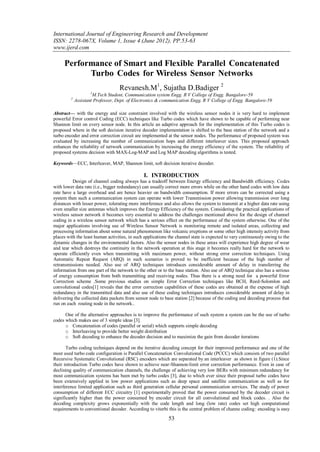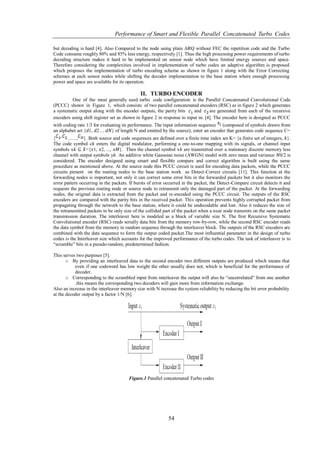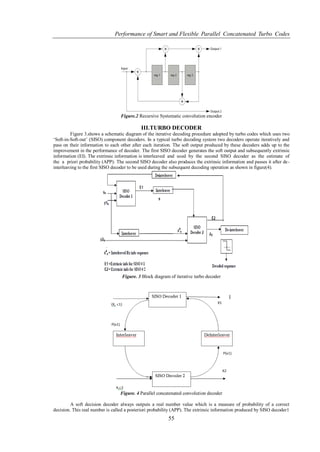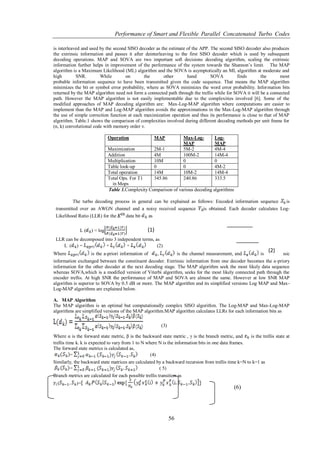Anúncio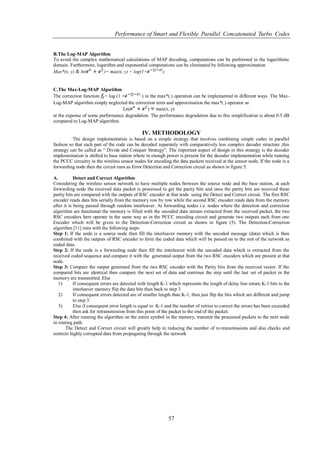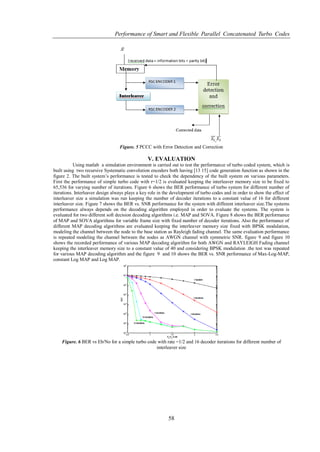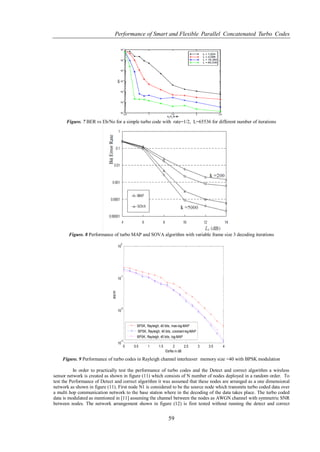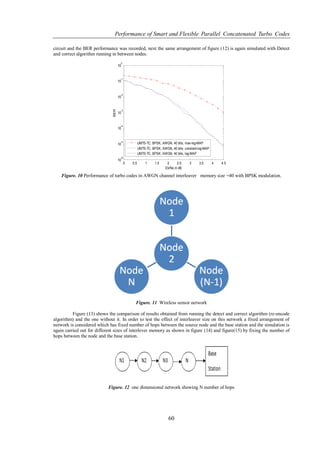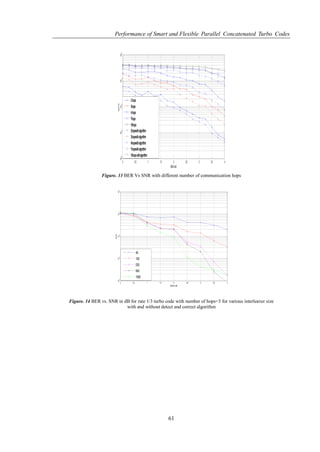Anúncio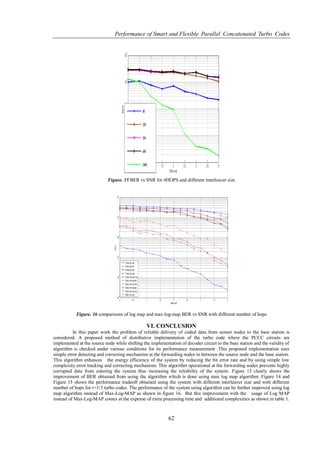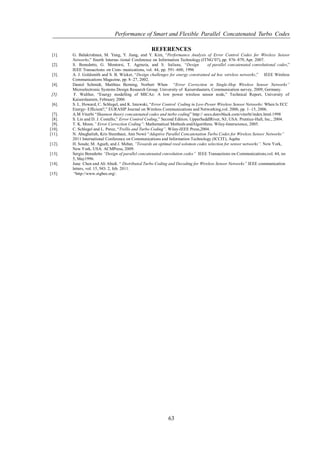Próximos SlideShares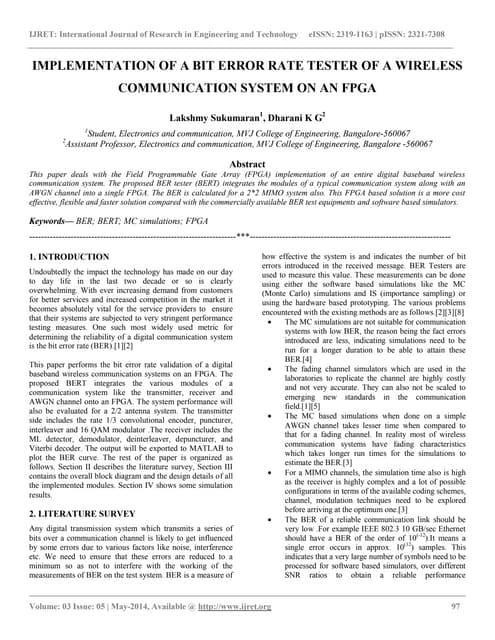Implementation of a bit error rate tester of a wireless communication system ...
Carregando em ... 3
1 de 11
Anúncio

### www.ijerd.com

1. International Journal of Engineering Research and Development ISSN: 2278-067X, Volume 1, Issue 4 (June 2012), PP.53-63 www.ijerd.com Performance of Smart and Flexible Parallel Concatenated Turbo Codes for Wireless Sensor Networks Revanesh.M1, Sujatha D.Badiger 2 1 M.Tech Student, Communication system Engg. R V College of Engg. Bangalore-59 2 Assistant Professor, Dept. of Electronics & communication Engg. R V College of Engg. Bangalore-59 Abstract— with the energy and size constraint involved with the wireless sensor nodes it is very hard to implement powerful Error control Coding (ECC) techniques like Turbo codes which have shown to be capable of performing near Shannon limit on every sensor node. In this article an adaptive approach for the implementation of this Turbo codes is proposed where in the soft decision iterative decoder implementation is shifted to the base station of the network and a turbo encoder and error correction circuit are implemented at the sensor nodes. The performance of proposed system was evaluated by increasing the number of communication hops and different interleaver sizes. This proposed approach enhances the reliability of network communication by increasing the energy efficiency of the system. The reliability of proposed systems decision with MAX-Log-MAP and Log MAP decoding algorithms is tested. Keywords—ECC, Interleaver, MAP, Shannon limit, soft decision iterative decoder. I. INTRODUCTION Design of channel coding always has a tradeoff between Energy efficiency and Bandwidth efficiency. Codes with lower data rate (i.e., bigger redundancy) can usually correct more errors while on the other hand codes with low data rate have a large overhead and are hence heavier on bandwidth consumption. If more errors can be corrected using a system then such a communication system can operate with lower Transmission power allowing transmission over long distances with lesser power, tolerating more interference and also allows the system to transmit at a higher data rate using even smaller size antennas which improves the Energy Efficiency of the system. Considering the practical applications of wireless sensor network it becomes very essential to address the challenges mentioned above for the design of channel coding in a wireless sensor network which has a serious effect on the performance of the system otherwise. One of the major applications involving use of Wireless Sensor Network is monitoring remote and isolated areas, collecting and processing information about some natural phenomenon like volcanic eruptions or some other high intensity activity from places with the least human activities, in such applications the channel state is expected to vary continuously owing to the dynamic changes in the environmental factors. Also the sensor nodes in these areas will experience high degree of wear and tear which destroys the continuity in the network operation at this stage it becomes really hard for the network to operate efficiently even when transmitting with maximum power, without strong error correction techniques. Using Automatic Repeat Request (ARQ) in such scenarios is proved to be inefficient because of the high number of retransmissions needed. Also use of ARQ techniques introduces considerable amount of delay in transferring the information from one part of the network to the other or to the base station. Also use of ARQ technique also has a serious of energy consumption from both transmitting and receiving nodes. Thus there is a strong need for a powerful Error Correction scheme .Some previous studies on simple Error Correction techniques like BCH, Reed-Solomlon and convolutional codes reveals that the error correction capabilities of these codes are obtained at the expense of high redundancy in the transmitted data and also use of these coding techniques introduces considerable amount of delay in delivering the collected data packets from sensor node to base station  because of the coding and decoding process that run on each routing node in the network.. One of the alternative approaches is to improve the performance of such system a system can be the use of turbo codes which makes use of 3 simple ideas . o Concatenation of codes (parallel or serial) which supports simple decoding o Interleaving to provide better weight distribution o Soft decoding to enhance the decoder decision and to maximize the gain from decoder iterations Turbo coding techniques depend on the iterative decoding concept for their improved performance and one of the most used turbo code configuration is Parallel Concatenation Convolutional Code (PCCC) which consists of two parallel Recursive Systematic Convolutional (RSC) encoders which are separated by an interleaver as shown in figure (1).Since their introduction Turbo codes have shown to achieve near-Shannon-limit error correction performance. Even in case of declining quality of communication channels, the challenge of achieving very low BERs with minimum redundancy for most communication systems has been met by turbo codes , due to which ever since their proposal turbo codes have been extensively applied in low power applications such as deep space and satellite communication as well as for interference limited application such as third generation cellular personal communication services. The study of power consumption of different ECC circuitry  experimentally proved that the power consumed by the decoder circuit is significantly higher than the power consumed by encoder circuit for all convolutional and block codes. . Also the decoding complexity grows exponentially with the code length and long (low rate) codes set high computational requirements to conventional decoder. According to viterbi this is the central problem of channe coding: encoding is easy 53
2. Performance of Smart and Flexible Parallel Concatenated Turbo Codes but decoding is hard . Also Compared to the node using plain ARQ without FEC the repetition code and the Turbo Code consume roughly 80% and 85% less energy, respectively . Thus the high processing power requirements of turbo decoding structure makes it hard to be implemented on sensor node which have limited energy sources and space. Therefore considering the complexities involved in implementation of turbo codes an adaptive algorithm is proposed which proposes the implementation of turbo encoding scheme as shown in figure 1 along with the Error Correcting schemes at each sensor nodes while shifting the decoder implementation to the base station where enough processing power and space are available for its operation. II. TURBO ENCODER One of the most generally used turbo code configuration is the Parallel Concatenated Convolutional Code (PCCC) shown in Figure 1, which consists of two parallel concatenated encoders (RSC) as in figure 2 which generates a systematic output along with the encoder outputs, the parity bits and are generated from each of the recursive encoders using shift register set as shown in figure 2 in response to input m. . The encoder here is designed as PCCC with coding rate 1/3 for evaluating its performance. The input information sequence (composed of symbols drawn from an alphabet set {𝑑1, 𝑑2 ... 𝑑𝑁} of length N and emitted by the source), enter an encoder that generates code sequence C= { ……, }. Both source and code sequences are defined over a finite time index set K= {a finite set of integers, 𝑘}. The code symbol c𝑘 enters the digital modulator, performing a one-to-one mapping with its signals, or channel input symbols x𝑘 ⊆ 𝑋={𝑥1, 𝑥2, ..., 𝑥𝑀}. Then the channel symbol x𝑘 are transmitted over a stationary discrete memory less channel with output symbols y𝑘. An additive white Gaussian noise (AWGN) model with zero mean and variance 𝑁0/2 is considered. The encoder designed using smart and flexible compare and correct algorithm is built using the same procedure as mentioned above. At the source node this PCCC circuit is used for encoding data packets, while the PCCC circuits present on the routing nodes to the base station work as Detect-Correct circuits . This function at the forwarding nodes is important, not only it can correct some error bits in the forwarded packets but it also monitors the error pattern occurring in the packets. If bursts of error occurred in the packet, the Detect-Compare circuit detects it and requests the previous routing node or source node to retransmit only the damaged part of the packet. At the forwarding nodes, the original data is extracted from the packet and re-encoded using the PCCC circuit. The outputs of the RSC encoders are compared with the parity bits in the received packet. This operation prevents highly corrupted packet from propagating through the network to the base station, where it could be undecodable and lost. Also it reduces the size of the retransmitted packets to be only size of the collided part of the packet when a near node transmits on the same packet transmission duration. The interleaver here is modeled as a block of variable size N. The first Recursive Systematic Convolutional encoder (RSC) reads serially data bits from the memory row-by-row, while the second RSC encoder reads the data symbol from the memory in random sequence through the interleaver block. The outputs of the RSC encoders are combined with the data sequence to form the output coded packet.The most influential parameter in the design of turbo codes is the Interleaver size which accounts for the improved performance of the turbo codes. The task of interleaver is to ―scramble‖ bits in a pseudo-random, predetermined fashion. This serves two purposes . o By providing an interleaved data to the second encoder two different outputs are produced which means that even if one codeword has low weight the other usually does not, which is beneficial for the performance of decoder. o Corresponding to the scrambled input from interleaver the output will also be ―uncorrelated‖ from one another .this means the corresponding two decoders will gain more from information exchange. Also an increase in the interleaver memory size with N increase the system reliability by reducing the bit error probability at the decoder output by a factor 1/N . Figure.1 Parallel concatenated Turbo codes 54
3. Performance of Smart and Flexible Parallel Concatenated Turbo Codes Figure.2 Recursive Systematic convolution encoder III.TURBO DECODER Figure 3.shows a schematic diagram of the iterative decoding procedure adopted by turbo codes which uses two ‗Soft-in-Soft-out‘ (SISO) component decoders. In a typical turbo decoding system two decoders operate iteratively and pass on their information to each other after each iteration. The soft output produced by these decoders adds up to the improvement in the performance of decoder. The first SISO decoder generates the soft output and subsequently extrinsic information (EI). The extrinsic information is interleaved and used by the second SISO decoder as the estimate of the a priori probability (APP). The second SISO decoder also produces the extrinsic information and passes it after de - interleaving to the first SISO decoder to be used during the subsequent decoding operation as shown in figure(4). Figure. 3 Block diagram of iterative turbo decoder Figure. 4 Parallel concatenated convolution decoder A soft decision decoder always outputs a real number value which is a measure of probability of a correct decision. This real number is called a posteriori probability (APP). The extrinsic information produced by SISO decoder1 55
4. Performance of Smart and Flexible Parallel Concatenated Turbo Codes is interleaved and used by the second SISO decoder as the estimate of the APP. The second SISO decoder also produces the extrinsic information and passes it after deinterleaving to the first SISO decoder which is used by subsequent decoding operations. MAP and SOVA are two important soft decisions decoding algorithm, scaling the extrinsic information further helps in improvement of the performance of the system towards the Shannon‘s limit. The MAP algorithm is a Maximum Likelihood (ML) algorithm and the SOVA is asymptotically an ML algorithm at moderate and high SNR. While on the other hand SOVA finds the most probable information sequence to have been transmitted given the code sequence. That means the MAP algorithm minimizes the bit or symbol error probability, where as SOVA minimizes the word error probability. Information bits returned by the MAP algorithm need not form a connected path through the trellis while for SOVA it will be a connected path. However the MAP algorithm is not easily implementable due to the complexities involved . Some of the modified approaches of MAP decoding algorithm are: Max-Log-MAP algorithm where computations are easier to implement than the MAP and Log-MAP algorithm avoids the approximations in the Max-Log-MAP algorithm through the use of simple correction function at each maximization operation and thus its performance is close to that of MAP algorithm. Table.1 shows the comparison of complexities involved during different decoding methods per unit frame for (n, k) convolutional code with memory order v. Operation MAP Max-Log- Log- MAP MAP Maximization 2M-1 5M-2 4M-4 Addition 4M 100M-2 14M-4 Multiplication 10M 0 0 Table look-up 0 0 4M-2 Total operation 14M 10M-2 14M-4 Total Ops. For T1 345.86 240.86 333.5 in Mops Table 1.Complexity Comparison of various decoding algorithms The turbo decoding process in general can be explained as follows: Encoded information sequence is transmitted over an AWGN channel and a noisy received sequence is obtained. Each decoder calculates Log- Likelihood Ratio (LLR) for the data bit as L( ) = log (1) LLR can be decomposed into 3 independent terms, as L ( )= + + (2) Where is the a-priori information of , is the channel measurement, and (2) is the extrinsic information exchanged between the constituent decoder. Extrinsic information from one decoder becomes the a-priory information for the other decoder at the next decoding stage. The MAP algorithm seek the most likely data sequence whereas SOVA,which is a modified version of Viterbi algorithm, seeks for the most likely connected path through the encoder trellis. At high SNR the performance of MAP and SOVA are almost the same. However at low SNR MAP algorithm is superior to SOVA by 0.5 dB or more. The MAP algorithm and its simplified versions Log MAP and Max- Log-MAP algorithms are explained below. A. MAP Algorithm The MAP algorithm is an optimal but computationally complex SISO algorithm. The Log-MAP and Max-Log-MAP algorithms are simplified versions of the MAP algorithm.MAP algorithm calculates LLRs for each information bits as (3) Where α is the forward state metric, β is the backward state metric , γ is the branch metric, and is the trellis state at trellis time k. k is expected to vary from 1 to N where N is the information bits in one data frames. The forward state metrics is calculated as, = (4) Similarly, the backward state matrices are calculated by a backward recursion from trellis time k=N to k=1 as = ( 5) Branch metrics are calculated for each possible trellis transition as ( )= (6) 56